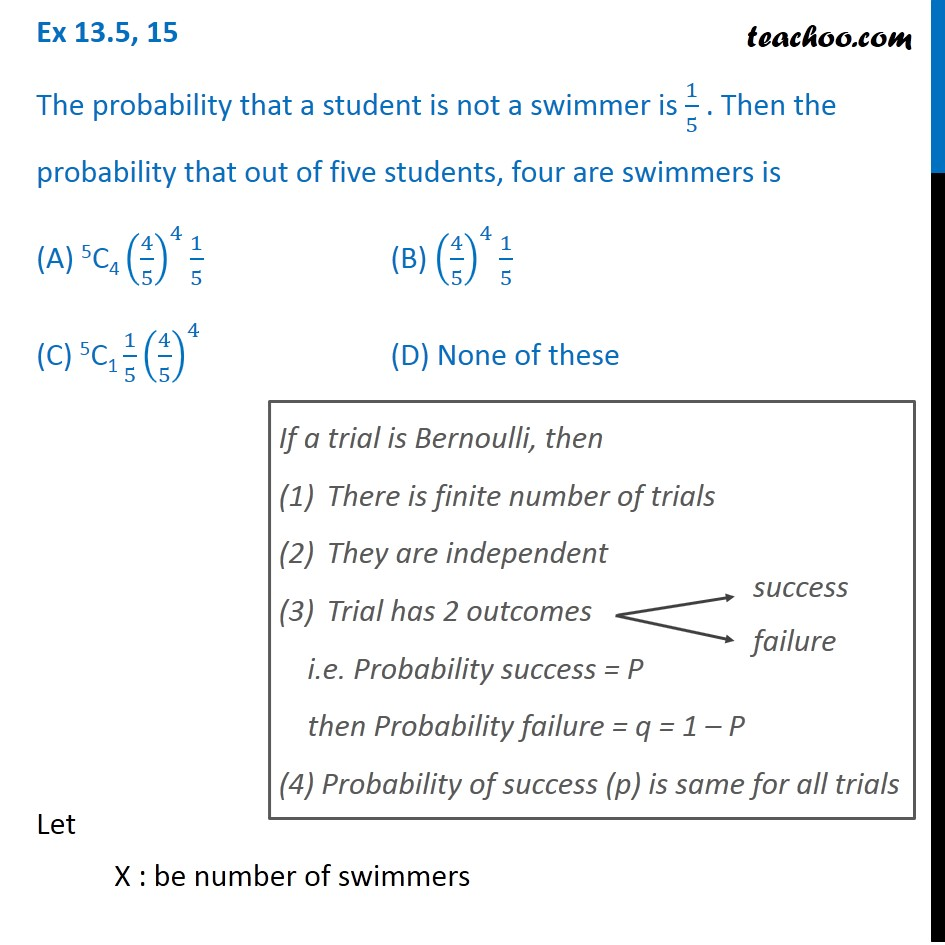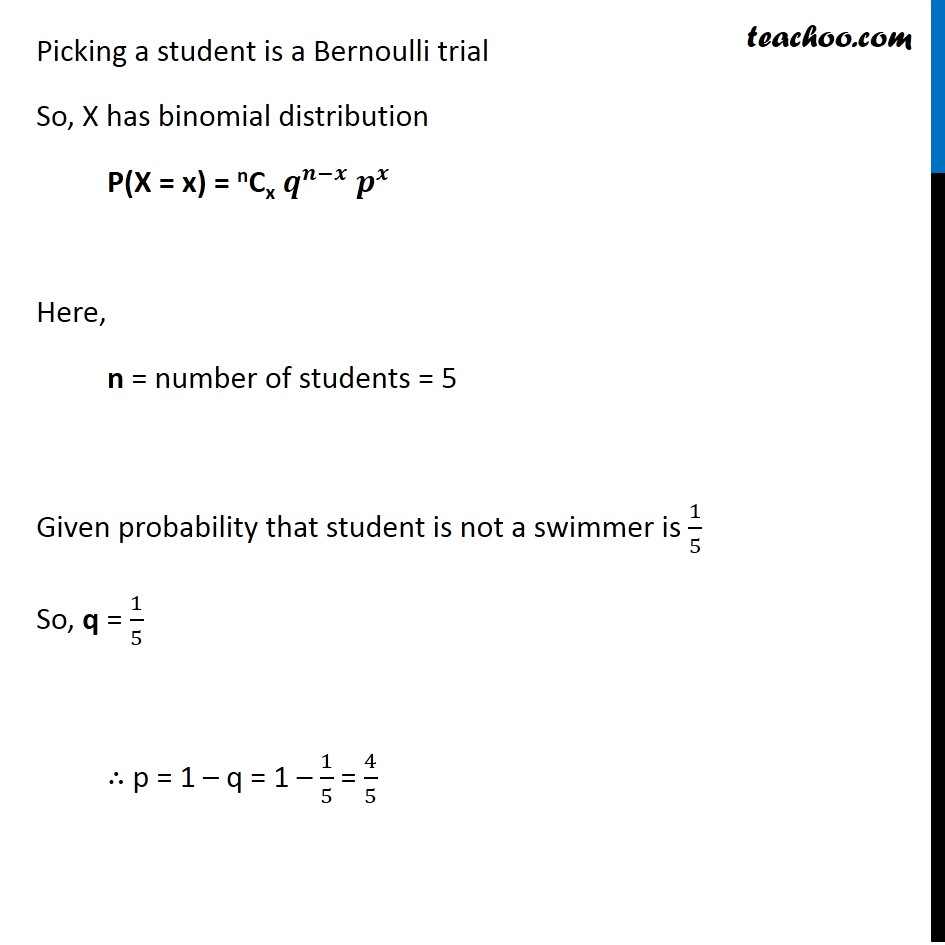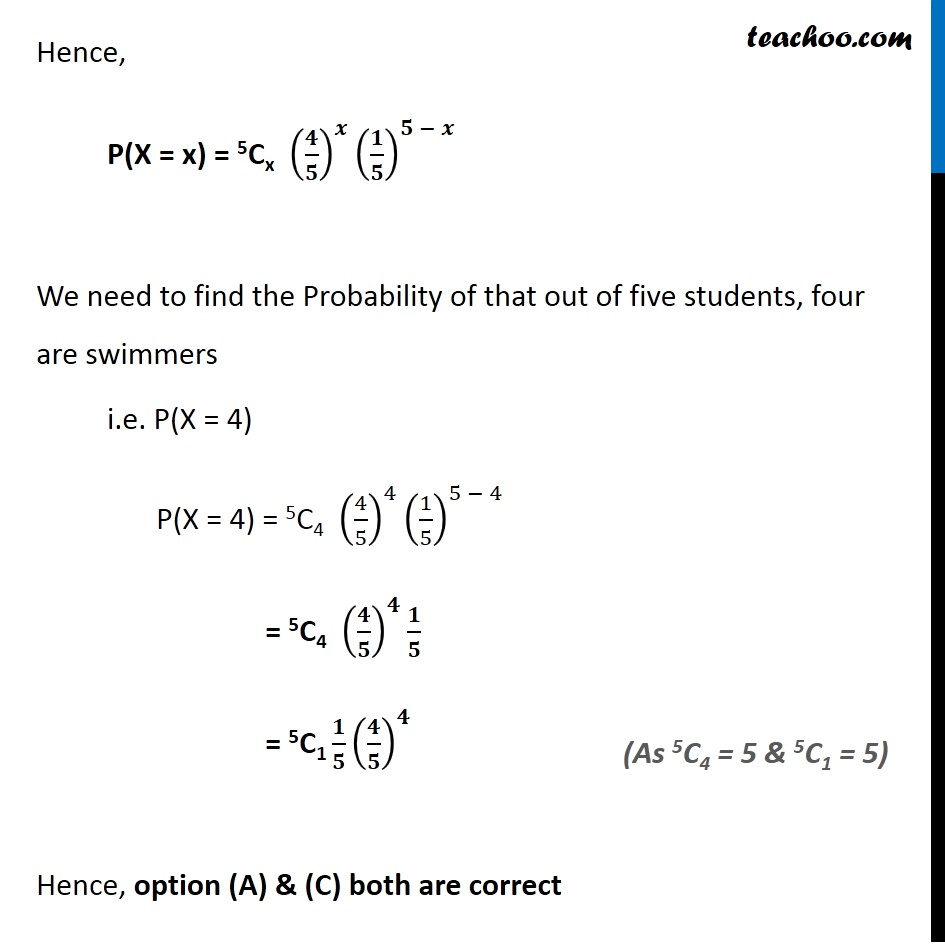Bernoulli Trial

Chapter 13 Class 12 Probability
Serial order wiseLearn in your speed, with individual attention - Teachoo Maths 1-on-1 Class

### Transcript

Question 15 The probability that a student is not a swimmer is 1/5 . Then the probability that out of five students, four are swimmers is (A) 5C4 (4/5)^4 1/5 (B) (4/5)^4 1/5 (C) 5C1 1/5 (4/5)^4 (D) None of theseIf a trial is Bernoulli, then There is finite number of trials They are independent Trial has 2 outcomes i.e. Probability success = P then Probability failure = q = 1 – P (4) Probability of success (p) is same for all trials Let X : be number of swimmers Picking a student is a Bernoulli trial So, X has binomial distribution P(X = x) = nCx 𝒒^(𝒏−𝒙) 𝒑^𝒙 Here, n = number of students = 5 Given probability that student is not a swimmer is 1/5 So, q = 1/5 ∴ p = 1 – q = 1 – 1/5 = 4/5 Hence, P(X = x) = 5Cx (𝟒/𝟓)^𝒙 (𝟏/𝟓)^(𝟓 − 𝒙) We need to find the Probability of that out of five students, four are swimmers i.e. P(X = 4) P(X = 4) = 5C4 (4/5)^4 (1/5)^(5 − 4) = 5C4 (𝟒/𝟓)^𝟒 𝟏/𝟓 = 5C1 𝟏/𝟓 (𝟒/𝟓)^𝟒 Hence, option (A) & (C) both are correct